# Basic Geometry : How to find the perimeter of a rectangle

## Example Questions

← Previous 1 3 4 5 6

### Example Question #1 : How To Find The Perimeter Of A Rectangle

Given the rectangle in the diagram, what is the perimeter of the rectangle?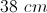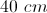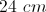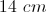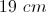Explanation:

The perimeter of a rectangle is found by adding up the length of all four sides. Since the two long sides are 12 cm, and the two shorter sides are 7 cm the perimeter can be found by: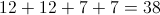The perimeter is 38 cm.

### Example Question #1 : How To Find The Perimeter Of A Rectangle

One side of a rectangle is 7 inches and another is 9 inches.  What is the perimeter of the rectangle in inches?Explanation:

To find the perimeter of a rectangle, add the lengths of the rectangle's four sides. If you have only the width and the height, then you can easily find all four sides (two sides are each equal to the height and the other two sides are equal to the width).  Multiply both the height and width by two and add the results.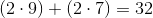### Example Question #1 : How To Find The Perimeter Of A Rectangle

A rectangle has an area of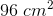. The width is four less than the length. What is the perimeter?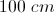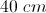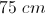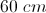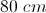Explanation:

For a rectangle, area is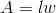and perimeter is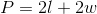, whereis the length and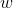is the width.

Let= length and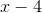= width.

The area equation to solve becomes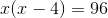, or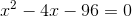.

To factor, find two numbers the sum to -4 and multiply to -96.  -12 and 8 will work:

x2 + 8x - 12x - 96 = 0

x(x + 8) - 12(x + 8) = 0

(x - 12)(x + 8) = 0

Set each factor equal to zero and solve: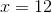or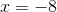.

Therefore the length is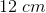and the width is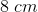, giving a perimeter of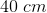.

### Example Question #2 : How To Find The Perimeter Of A Rectangle

A rancher wants to surround his rectangular field with barbed wire fence that costs \$1.75 per foot. The field measures 200 yards by 400 yards. How much will the fence cost?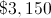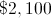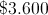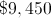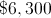Explanation:

Convert the dimensions from yards to feet by multiplying by 3: This makes the dimensions 600 feet and 1,200 feet. The perimeter of this farm is therefore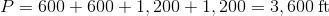.

Multiply this by the cost of the fence per foot: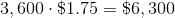### Example Question #5 : How To Find The Perimeter Of A Rectangle

A rectangle has a length ofinches and a width ofinches, find the perimeter of the rectangle.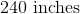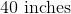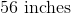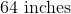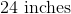Explanation:

To find the perimeter of any rectangle, add all of the sides up:

20 + 20 + 12 + 12 = 64 inches

You could use this formula as well:, where P = perimeter,  l = length, w = width

This formula comes from the fact that there are 2 lengths and 2 widths in every rectangle.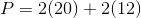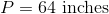### Example Question #1 : How To Find The Perimeter Of A Rectangle

Find the perimeter of this rectangle.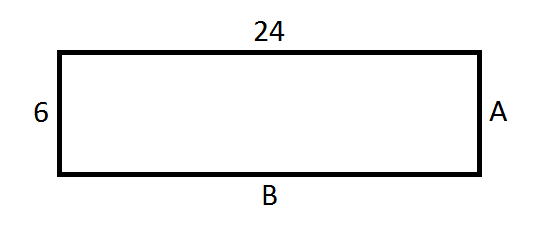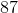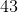Explanation:

Remember, the perimeter of the rectangle is the sum of all four sides: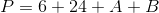It is obvious from the figure that A=6 and B=24.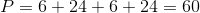### Example Question #1 : How To Find The Perimeter Of A Rectangle

A rectangle has an area of 56 square feet, and a width of 4 feet. What is the perimeter, in feet, of the rectangle?

36
14
120
30
28
Explanation:

Divide the area of the rectangle by the width in order to find the length of 14 feet. The perimeter is the sum of the side lengths, which in this case is 14 feet + 4 feet +14 feet + 4 feet, or 36 feet.

### Example Question #8 : How To Find The Perimeter Of A Rectangle

The area of a rectangle is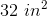, and the width of this rectangle is two times its height.  What is the perimeter of the rectangle?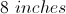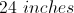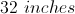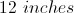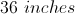Explanation:

The area of a rectangle is the width times the height, and we are told that in this rectangle the width is two times the height.

Therefore,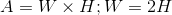.

Plug in the value of the area: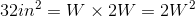Solve for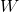to find a width of 4 inches. Using our formula above, the height must be 8 inches.  We then add all the sides of the rectangle together to find the perimeter: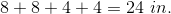### Example Question #1 : How To Find The Perimeter Of A Rectangle

Robert is designing a rectangular garden. He wants the area of the garden to be 9 square meters. If the length of the lot is going to be three meters less than twice the width, what will the perimeter of the lot be in meters?

1.5

10

3

6

12

12

Explanation:

Let l be the length of the garden and w be the width.

By the specifications of the problem, l = 2w-3.

Plug this in for length in the area formula:

A = l  x w = (2w - 3) x w = 9

Solve for the width:

2w²- 3w - 9 =0

(2w + 3)(w - 3) = 0

w is either 3 or -3/2, but we can't have a negative width, so w = 3.

If w = 3, then length = 2(3) - 3 = 3.

Now plug the width and length into the formula for perimeter:

P = 2 l + 2w = 2(3) + 2(3) = 12

### Example Question #1 : How To Find The Perimeter Of A Rectangle

Find the perimeter of a rectangle that has a length ofand a width of.Explanation:

Recall how to find the perimeter of a rectangle: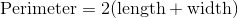For the given rectangle,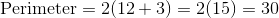← Previous 1 3 4 5 6